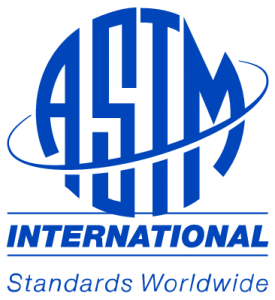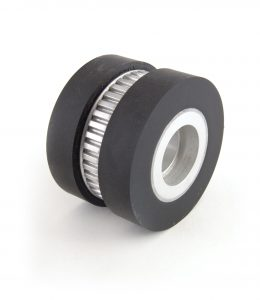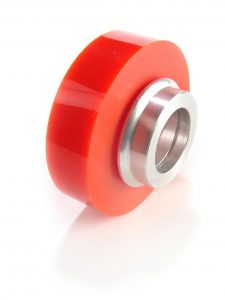# Directory of ASTM Test Methods for Cast Urethane Parts

### Want to know how to test Polyurethane physical properties as defined by ASTM? You’ve come to the right place!

Polyurethane is very similar to a rubber or plastic. Unlike nylon or UHMW, urethane can be compounded to produce very soft parts, as low as 20A Durometer, or up to very hard, 75D Durometer. Polyurethane is ideal in high demand applications were normal rubber would fail. The directory of test methods for cast urethane parts that are specified by ASTM are (a specific test method could vary):

1. Tensile Strength:  (ASTM D412) The tensile strength of a material is the maximum amount of tensile stress that it can be subjected to before failure. The definition of failure can vary according to material type and design methodology. This is an important concept in engineering, especially in the fields of material science, mechanical engineering and structural engineering.
2. Tear Strength:  (ASTM D624) Tear resistance (tear strength) is a measure of how well a material can withstand the effects of tearing. It is a useful engineering measurement for a wide variety of materials by many different test methods.3. Durometer Hardness:  (ASTM D2240)  Durometer is one of the several measures of the hardness of a material. Higher numbers indicate harder materials; lower numbers indicate softer materials.
4. Tension:  (ASTM D412) Tension strength is commonly measured by pounds per inch in America. For example, rubber has a tensile strength of 2030 psi while comparable hardness urethane has a 3250 psi.
5. Compression Set:  (ASTM D395) The compression set of a material is the permanent deformation remaining when a force that was applied to it) is removed. The term is normally applied to soft materials such as elastomers. There are two ways this is normally measured; compression set A and compression set B.
6. Resilience by Verticle Rebound:  (ASTM D2632) Resilience of a rubber compound is a measurement of how elastic it is when exposed to various stresses. Measurement of a material’s resilience can assist engineers and scientists with choosing the right material for a given application.7. Elongation: (ASTM D412) Elongation is defined as the length at breaking point expressed as a percentage of its original length (i.e. length at rest) e.g. if a urethane reaches twice its length before breaking its elongation is 100%. Specialty urethane parts have a 300% elongation rating for special applications.
8. Coefficient of Friction: (ASTM D1894)  A measure of the amount of resistance that a surface exerts on or substances moving over it, equal to the ratio between the maximal frictional force that the surface exerts and the force pushing the object toward the surface.
9. Specific Gravity: (ASTM D792) Specific gravity is the ratio of the density of a substance to the density of a reference substance; equivalently, it is the ratio of the mass of a substance to the mass of a reference substance for the same given volume.
10. Static Electrification: (ASTM D4470) Tendency of insulators to accumulate an electric charge, esp when rubbed by another material. High voltages can be generated leading to a spark discharge which may be hazardous.As an example, the Summary of ASTM Test Method – D2240 for hardness is: This test method permits hardness measurements based on either initial indentation or indentation after a specified period of time or both. Durometers with maximum reading indicators used to determine maximum hardness values of a material may yield lower hardness when the maximum indicator is used.

Before processing polyurethane there are several tests that can be performed. The tests aid in quality control and the elimination of producing out of spec parts. The industry utilizes the following polyurethane raw material tests:

1. Moisture – Karl-Fischer (ASTM D4672)
2. Viscosity – Brookfield (ASTM D445)
3. Color – Alpha (ASTM D1209)
4. %NCO – (ASTM D2572)
5. Melting Point – (ASTM D1519)